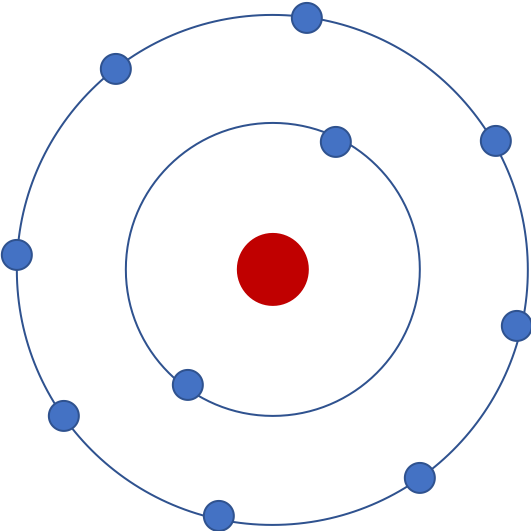Preview - Animal Behavior Lab (Isopods)

## Using models to learn science

Scientists use scientific modeling approaches to construct knowledge about the world. In this section, we explore the ideas behind scientific models.

### Questions

It only became widely accepted knowledge that all matter in the world is made up of tiny elementary particles in the early 19th century.

Let's look at the the picture below. What do you think this image is a model of?Some of you probably said it's a model of an atom. Others might say it's a model of a 'Neon atom', because it has 10 electrons. In fact, since we don't know the number of protons, it could be an ion of a different element!

The point is that these representations in a model allow us to think about natural phenomena (like atoms containing electrons) that are associated with the model in certain way. Can you think of what this particular model could be useful for?

Now, let's look at a computational model of a forest. Imagine that you have a drone with a camera that is hovering above a forest. In other words, this model shows a top-down view of a forest. Each green patch you see represents a tree. A red patch represents a burning tree.

Play with the model and make some observations.

What do you think a researcher or scientist could use this model for?

Make sure to change the density of trees in the model and observe the spread of the fire.

In this model, trees are called agents because their behaviors are programmed into the model using a set of rules.

An example of one such rule is a tree cannot move. Another is when a tree is on fire, it turns red.

How might you write a rule for a tree to model the spread of fire?

Based on your exploration of the model, can you guess how the density of trees affects the spread of the fire in the forest?

This 'fire model' is an example of an emergent systems microworld. It is modeled in terms of interactions between the agents (trees) and it allows us to observe emergent patterns like the spread of fire in the forest. Because it is a computational model, we can easily change parameters such as the density of trees and then study how that change affects the spread of fire in the forest. Although this is just a model, we can use that knowledge to make predictions regarding the spread of fire in a real forest.

However, this model does not include all the factors that affect the spread of fire in a real forest. Can you suggest some other factors that might affect the spread of a real forest fire and that could be added in this model?

### Notes

These notes will appear on every page in this lesson so feel free to put anything here you'd like to keep track of.